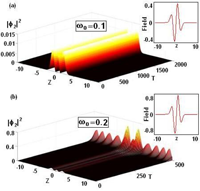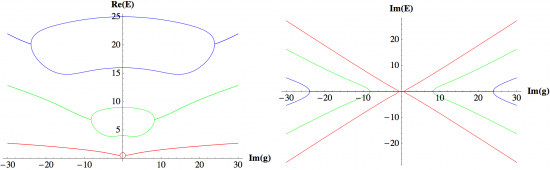September 2010
Mon Tue Wed Thu Fri Sat Sun
Oct »
12345
6789101112
13141516171819
20212223242526
27282930

## Supersymmetric extension of non-Hermitian su(2) Hamiltonians and supercoherent states

O. Cherbal, M. Drir, M. Maamache, D.A. Trifonov

A new class of non-Hermitian Hamiltonians with real spectrum, which are written as a real linear combination of su(2) generators is analyzed. The metric which allows the transition to the equivalent Hermitian Hamiltonian is established. A pseudo-Hermitian supersymmetic extension of such Hamiltonians is performed, which correspond to the pseudo-Hermitian supersymmetric system of the boson-phermion oscillator. We extend the supercoherent states formalism to such supersymmetic systems via the pseudo-unitary supersymmetric displacement operator method. The constructed family of these supercoherent states consists of two dual subfamilies that form a bi-overcomplete and bi-normal system in the boson-phermion Fock space. The states of each subfamily are eigenvectors of the boson annihilation operator and of one of the two phermion lowering operators.

http://arxiv.org/abs/1009.5293
Quantum Physics (quant-ph)

## Spontaneous Parity–Time Symmetry Breaking and Stability of Solitons in Bose-Einstein Condensates

Zhenya Yan, Bo Xiong, Wu-Ming LiuWe report explicitly a novel family of exact PT-symmetric solitons and further study their spontaneous PT symmetry breaking, stabilities and collisions in Bose-Einstein condensates trapped in a PT-symmetric harmonic trap and a Hermite-Gaussian gain/loss potential. We observe the significant effects of mean-field interaction by modifying the threshold point of spontaneous PT symmetry breaking in Bose-Einstein condensates. Our scenario provides a promising approach to study PT-related universal behaviors in non-Hermitian quantum system based on the manipulation of gain/loss potential in Bose-Einstein condensates.

http://arxiv.org/abs/1009.4023
Quantum Gases (cond-mat.quant-gas)

## Extending PT symmetry from Heisenberg algebra to E2 algebra

Carl M. Bender, R. J. KalveksThe E2 algebra has three elements, J, u, and v, which satisfy the commutation relations [u,J]=iv, [v,J]=-iu, [u,v]=0. We can construct the Hamiltonian H=J^2+gu, where g is a real parameter, from these elements. This Hamiltonian is Hermitian and consequently it has real eigenvalues. However, we can also construct the PT-symmetric and non-Hermitian Hamiltonian H=J^2+igu, where again g is real. As in the case of PT-symmetric Hamiltonians constructed from the elements x and p of the Heisenberg algebra, there are two regions in parameter space for this PT-symmetric Hamiltonian, a region of unbroken PT symmetry in which all the eigenvalues are real and a region of broken PT symmetry in which some of the eigenvalues are complex. The two regions are separated by a critical value of g.

http://arxiv.org/abs/1009.3236
High Energy Physics – Theory (hep-th); Mathematical Physics (math-ph); Quantum Physics (quant-ph)

## PT Symmetry and the Sign Problem

Peter N. Meisinger, Michael C. Ogilvie, Timothy D. WiserGeneralized PT symmetry provides crucial insight into the sign problem for two classes of models. In the case of quantum statistical models at non-zero chemical potential, the free energy density is directly related to the ground state energy of a non-Hermitian, but generalized PT-symmetric Hamiltonian. There is a corresponding class of PT-symmetric classical statistical mechanics models with non-Hermitian transfer matrices. For both quantum and classical models, the class of models with generalized PT symmetry is precisely the class where the complex weight problem can be reduced to real weights, i.e., a sign problem. The spatial two-point functions of such models can exhibit three different behaviors: exponential decay, oscillatory decay, and periodic behavior. The latter two regions are associated with PT symmetry breaking, where a Hamiltonian or transfer matrix has complex conjugate pairs of eigenvalues. The transition to a spatially modulated phase is associated with PT symmetry breaking of the ground state, and is generically a first-order transition. In the region where PT symmetry is unbroken, the sign problem can always be solved in principle. Moreover, there are models with PT symmetry which can be simulated for all parameter values, including cases where PT symmetry is broken.

http://arxiv.org/abs/1009.0745
High Energy Physics – Theory (hep-th)

## Automatic Hermiticity

Keiichi Nagao and Holger Bech Nielsen

We study the Hamiltonian that is not at first hermitian. Requirement that a measurement shall not change one Hamiltonian eigenstate into another one with a different eigenvalue imposes that an inner product must be defined so as to make the Hamiltonian normal with regard to it. After a long time development with the non-hermitian Hamiltonian, only a subspace of possible states will effectively survive. On this subspace the effect of the anti-hermitian part of the Hamiltonian is suppressed, and the Hamiltonian becomes hermitian. Thus hermiticity emerges automatically, and we have no reason to maintain that at the fundamental level the Hamiltonian should be hermitian. We also point out a possible misestimation of a past state by extrapolating back in time with the hermitian Hamiltonian. It is a seeming past state, not a true one.

http://arxiv.org/abs/1009.0441
Quantum Physics (quant-ph); High Energy Physics – Theory (hep-th); General Physics (physics.gen-ph)